## VERTEX FORM OF A QUADRATIC FUNCTION WORKSHEET

1. Write the quadratic function in vertex form whose graph is shown below.Questions 2-4 : Write the quadratic function in vertex form and write its vertex.

2. f(x) = x 2  + 6x + 9

3. f(x) = -x 2  - 2x - 2

4. f(x) = 4x 2  + 16x + 5

5.  Write the following quadratic function in vertex form and sketch its graph :

f(x) = -x 2  + 2 x + 3The parabola above opens up with vertex (2, 1).

Vertex form of a quadratic function :

f(x) = a(x - h) 2 + k

Substitute vertex (h, k) = (2, 1).

f(x) = a(x - 2) 2 + 1 ----(1)

The y-intercept of the parabola is (0, 5). That is, the parabola is passing through (0, 5).

Substitute x = 0 and y = 5.

5 = a(0 - 2) 2 + 1

5 = a(- 2) 2  + 1

5 = 4a + 1

Subtract 1 from both sides.

Divide both sides by 4.

Substitute a = 1 in (1).

f(x) = 1(x - 2) 2  + 1

Vertex form of the quadratic function :

f(x) = x 2  + 6x + 9

f(x) = x 2  + 2(x)(3) + 3 2

f(x) = (x + 3) 2

Comparing  y = a(x - h) 2  + k and  f(x) = (x + 3) 2 ,

h = -3 and k = 0

So, the vertex is (h, k) = (-3, 0).

f(x) = -x 2  - 2 x - 2

f(x) = -(x 2  + 2x) - 2

f(x) = -[x 2  + 2(x)(1) + 1 2  - 1 2 ] - 2

f(x) = -[(x + 1) 2  - 1 2 ] - 2

f(x) = -[(x + 1) 2  - 1] - 2

f(x) = -(x + 1) 2  + 1 - 2

f(x) = -(x + 1) 2  - 1

Comparing f(x)  = a(x - h) 2  + k and f(x) = -(x + 1) 2  - 1,

h = -1 and k = -1

So, the vertex is (h, k) = (-1, -1).

f(x) = 4x 2  + 16x + 5

f(x) = 4(x 2  + 4x) + 5

f(x) = 4[x 2  + 2(x)(2) + 2 2  - 2 2 ] + 5

f(x) = 4[(x + 2) 2  - 2 2 ] + 5

f(x) = 4[(x + 2) 2  - 4] + 5

f(x) = 4(x + 2) 2  - 16 + 5

f(x) = 4(x + 2) 2  - 11

Comparing f(x)  = a(x - h) 2  + k and f(x) = 4(x + 2) 2  - 11,

h = -2 and k = -11

So, the vertex is (h, k) = (-2, -11).

Vertex form of the quadratic equation :

f(x) = -(x 2  - 2x) + 3

f(x) = -[x 2  - 2(x)(1) + 1 2  - 1 2 ] + 3

f(x) = -[(x - 1) 2  - 1 2 ] + 3

f(x) = -[(x - 1) 2  - 1] + 3

f(x) = -(x - 1) 2  + 1 + 3

f(x) = -(x - 1) 2  + 4

Comparing  f(x)  = a(x - h) 2  + k and  f(x)  = -(x - 1) 2  + 4,

h = 1 and k = 4

So, the vertex is (h, k) = (1, 4).

To graph the above quadratic equation, we need to find x-intercept and y-intercept, if any.

x-intercept :

To find the x-intercept, substitute f(x) = 0.

0 = -(x - 1) 2  + 4

(x - 1) 2  = 4

Take square root on both sides.

x - 1 = ±√4

x - 1 =  ±2

x - 1 = -2  or  x - 1 = 2

x = -1  or  x = 3

So, the x-intercepts are (-1, 0) and (3, 0) .

y-intercept :

To find the y-intercept, substitute x = 0.

f(0) = -(0 - 1) 2  + 4

= -(-1) 2  + 4

= -1 + 4

So, the y-intercept is (0, 3).

Comparing f(x)  = a(x - h) 2  + k and  f(x)  = -(x - 1) 2  + 4,

So, the parabola opens down with vertex (1, 4), x-intercepts (-1, 0) and (3, 0) and y-intercept (0, 3).Kindly mail your feedback to   [email protected]

• Sat Math Practice
• SAT Math Worksheets
• PEMDAS Rule
• BODMAS rule
• GEMDAS Order of Operations
• Math Calculators
• Transformations of Functions
• Order of rotational symmetry
• Lines of symmetry
• Compound Angles
• Quantitative Aptitude Tricks
• Trigonometric ratio table
• Word Problems
• Times Table Shortcuts
• 10th CBSE solution
• PSAT Math Preparation
• Laws of Exponents## Recent Articles## Endpoints of Diameter of a Circle

Nov 26, 23 07:28 AM## Solving Difficult Problems Using Elimination Method

Nov 25, 23 01:52 AM## Elimination Method Examples

Nov 24, 23 07:35 AM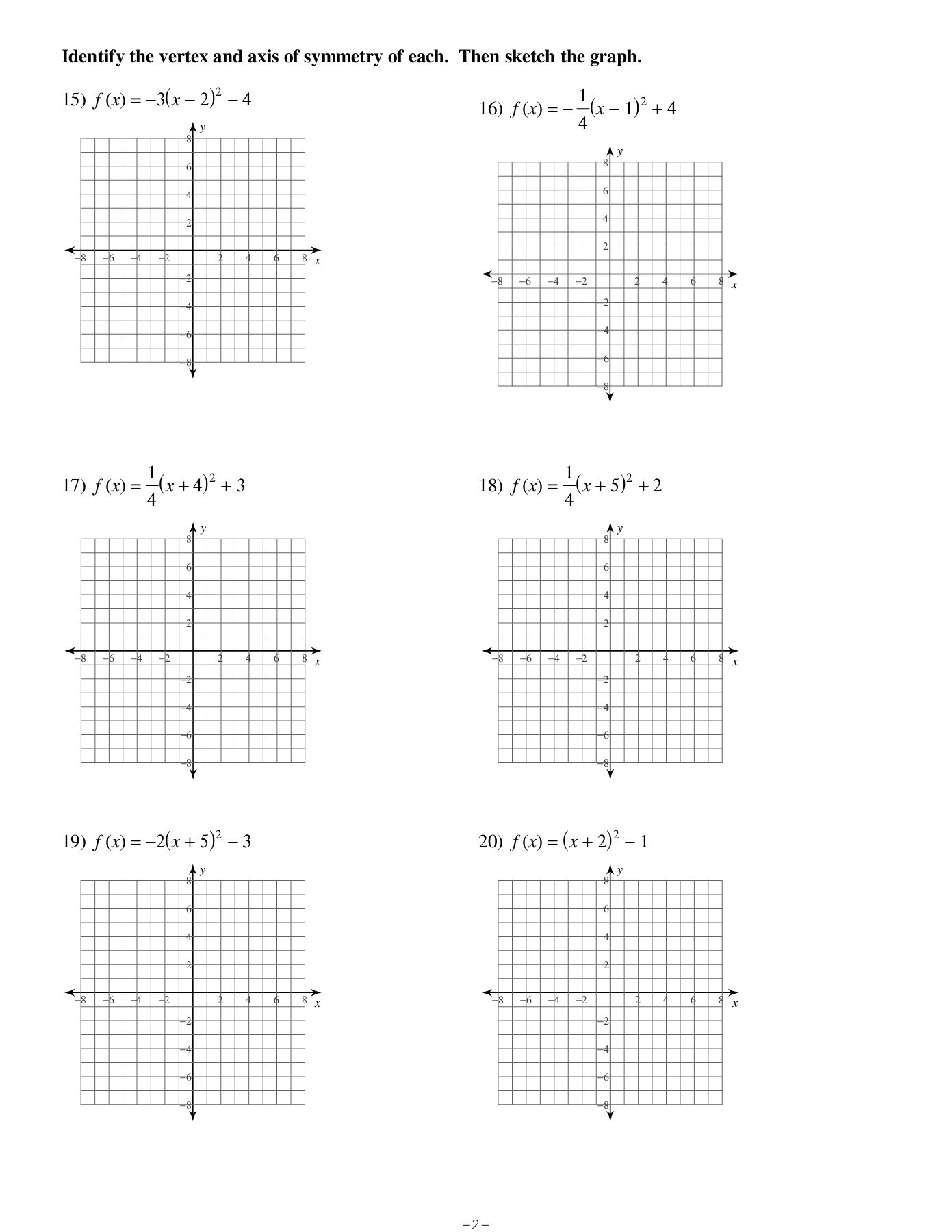## Practice Worksheet Graphing Quadratics In Vertex Form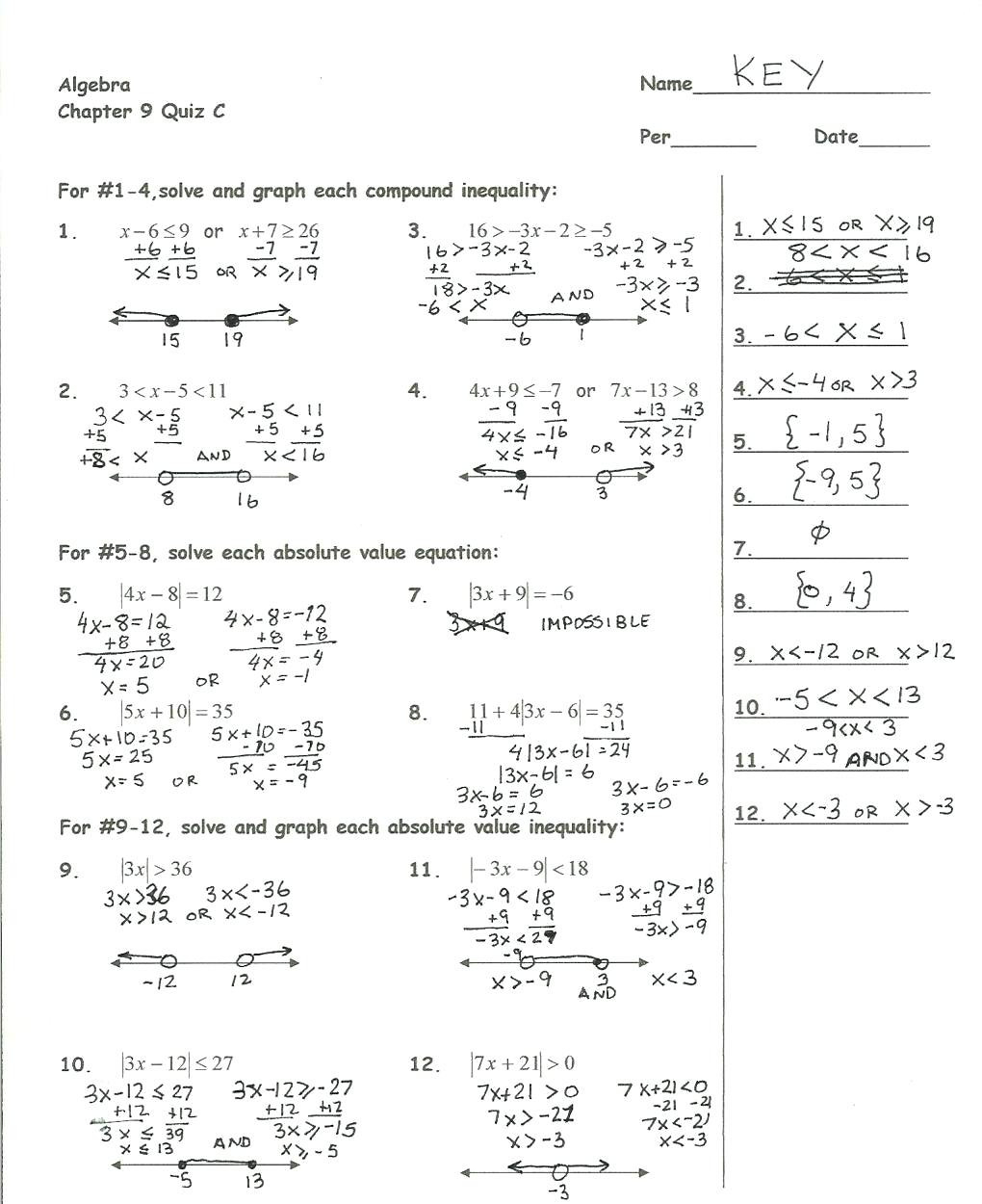A quadratic worksheet will also help you learn how to find the sum, product, and discriminant of quadratic equations. Students will use the free printable worksheets to solve quadratic equations and practice identifying the nature and number of roots. The worksheets also teach students how to solve MCQs.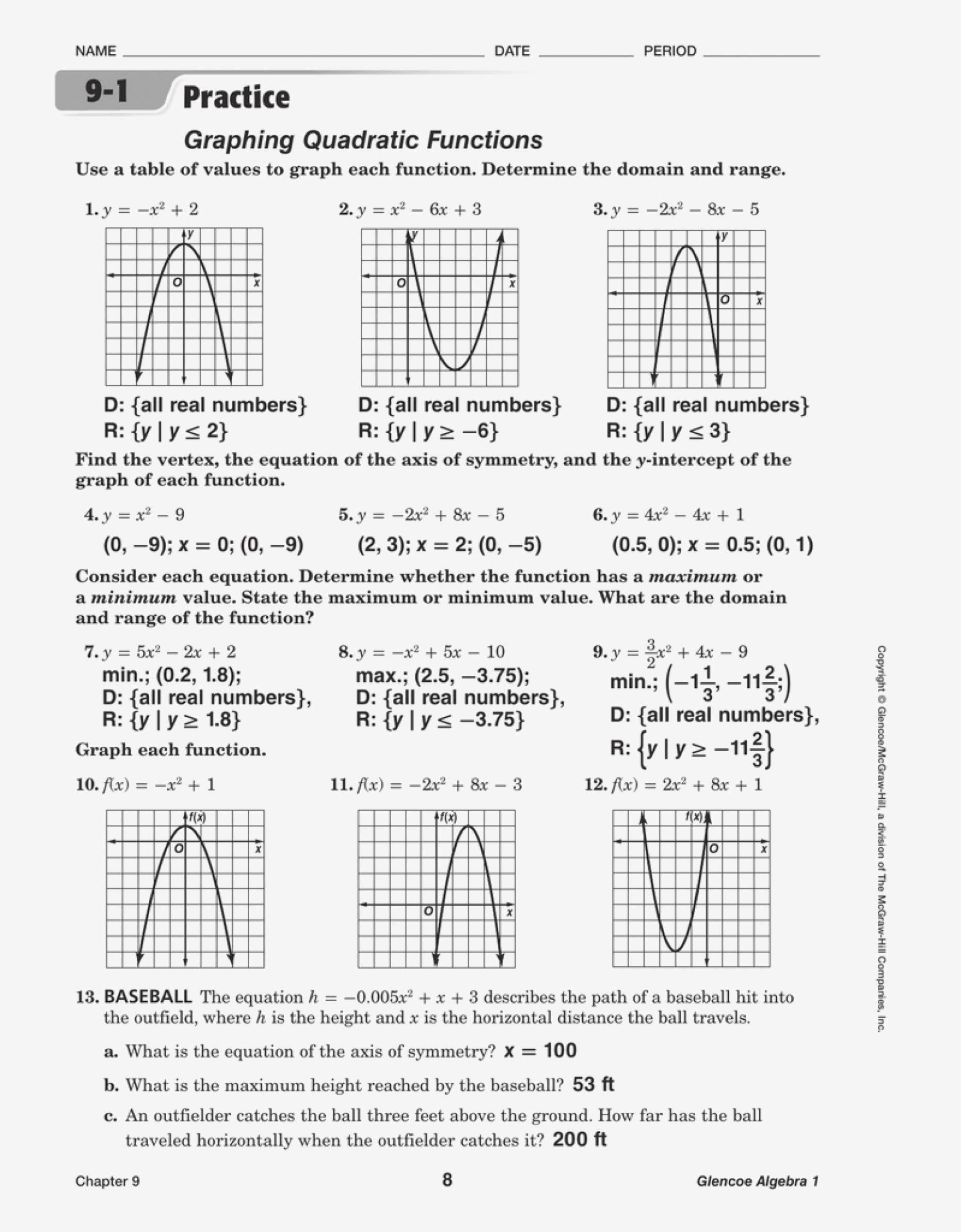Quadratic worksheets will aid students in developing a solid understanding and confidence in solving quadratic equations. This worksheet is great for reviewing what students have learned and to evaluate their progress. In addition, the worksheet will help students become more independent as they get more practice solving quadratic equations.## Gallery of Practice Worksheet Graphing Quadratics In Vertex FormSave my name, email, and website in this browser for the next time I comment.#### IMAGES

1. 40 graphing quadratics in vertex form worksheet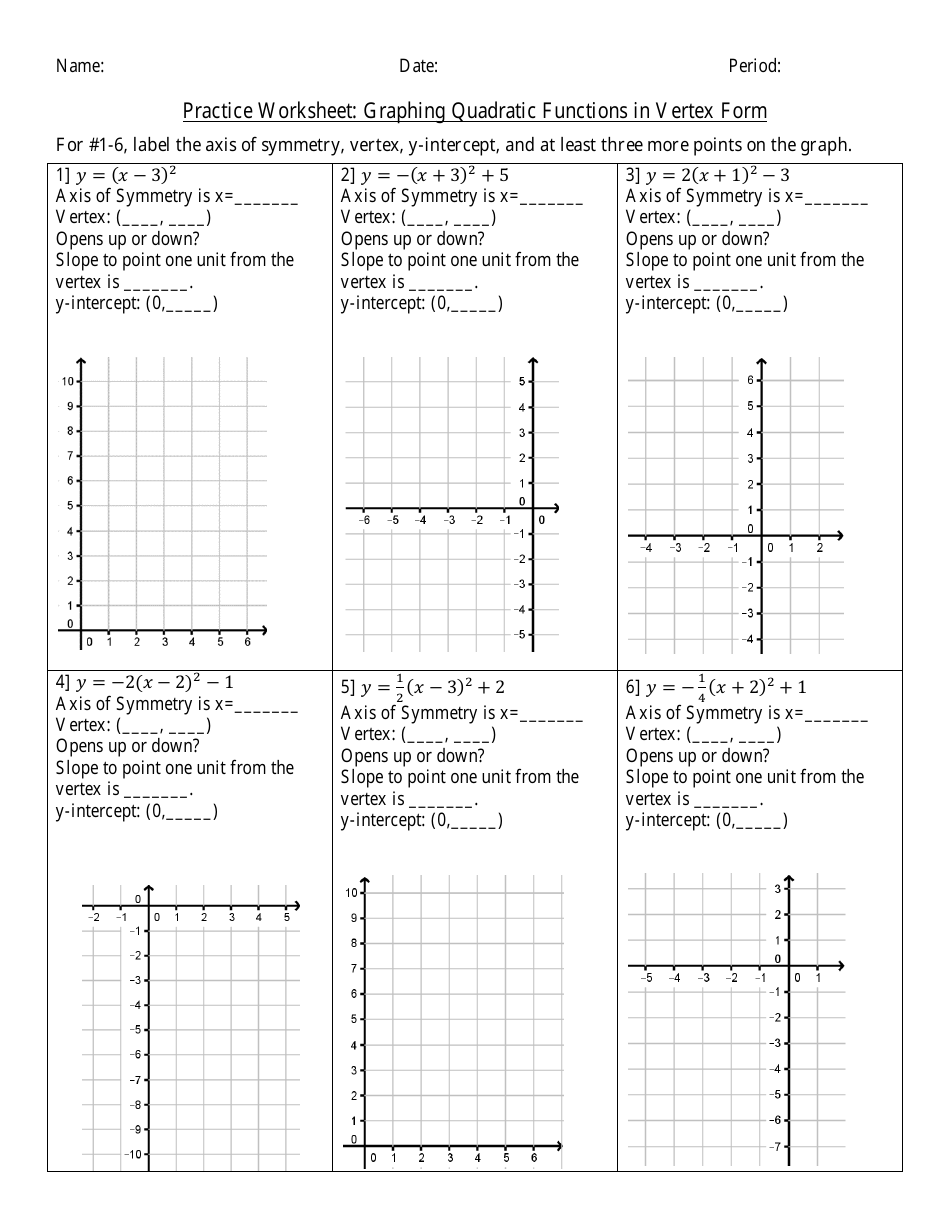2. Practice Worksheet Graphing Quadratic Functions In Standard Form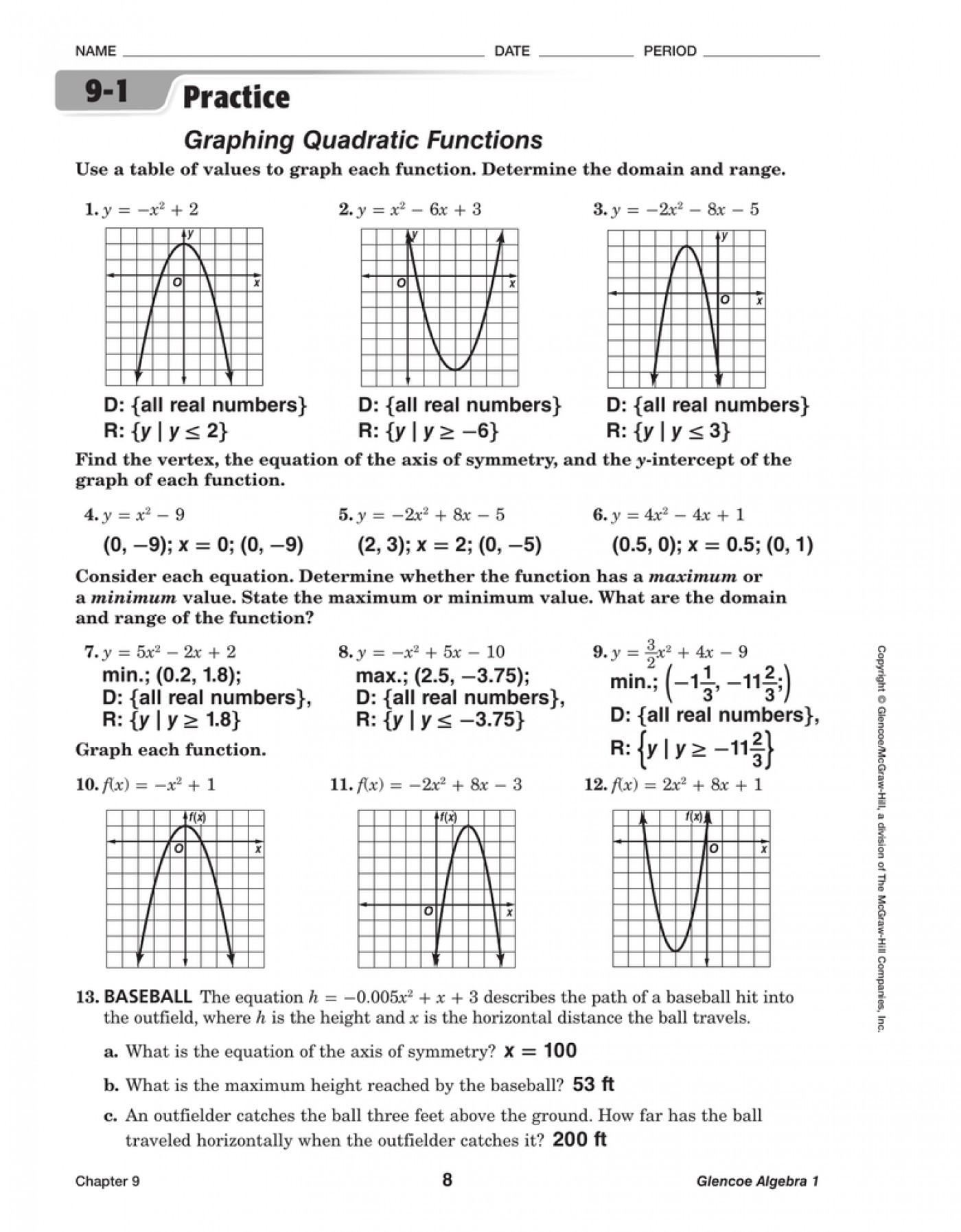3. Review solving quadratics by graphing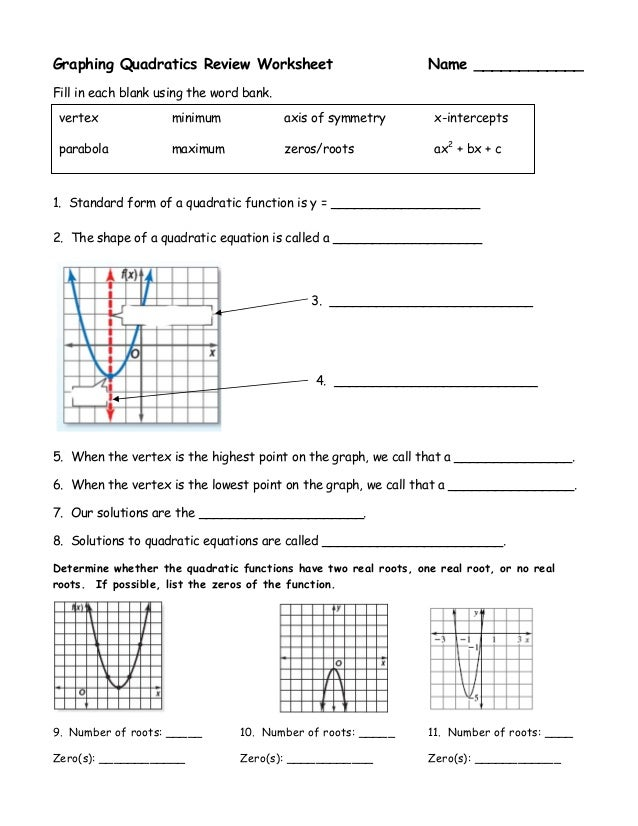4. graphing quadratics in vertex form worksheet5. Graphing Quadratics in Vertex Form (Practice Worksheet) by Lisa Davenport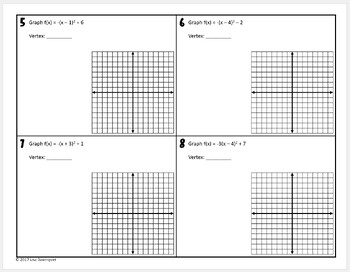6. Practice Worksheet Graphing Quadratic Functions In Vertex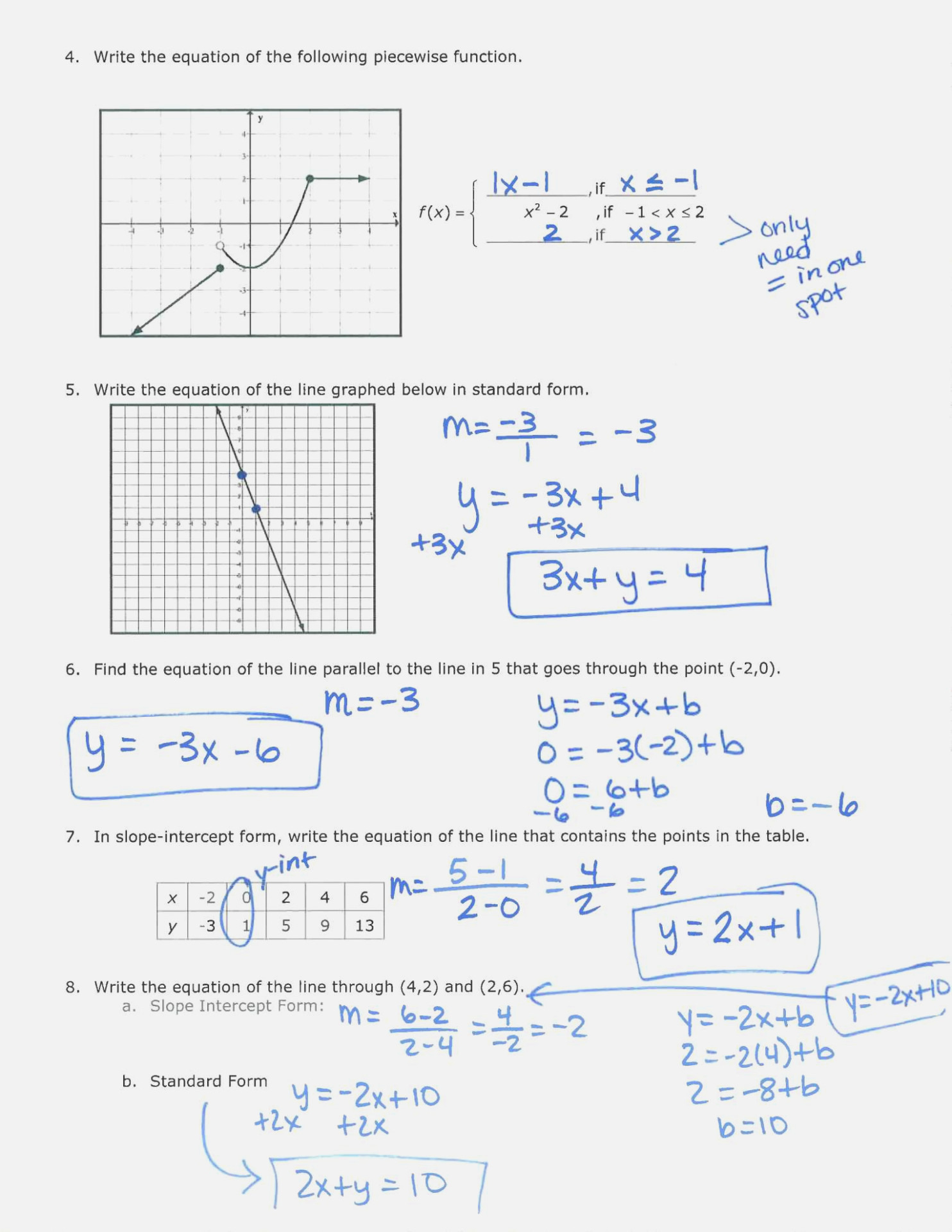#### VIDEO

1. Graphing quadratics from Vertex From

4. How to Graph Vertex Form Quadratics

6. Graphing Quadratics Vertex and Factored Form

Algebra 1 Course: Algebra 1 > Unit 14 Vertex form introduction Math > Algebra 1 > Quadratic functions & equations Graph quadratics in vertex form Google Classroom You might need: Calculator Graph the function. g ( x) = 1 3 ( x − 6) 2 + 1 1 2 3 4 5 6 7 8 9 − 2 − 3 − 4 − 5 − 6 − 7 − 8 − 9 1 2 3 4 5 6 7 8 9 − 2 − 3 − 4 − 5 − 6 − 7 − 8 − 9 y x

2. PDF HW

HW - Graphing Quadratic Functions in Vertex Form Sketch the graph of each function. y = -x2 vertex: _________ a=______ domain: ____________ range: ___________ axis of symmetry: _____________ increasing on: __________ decreasing on: __________ 3) y = (x - 1)2 2 -2 -1 2) y = (x - 2)2 - 3 2 1.5 0.5 -0.5 -1 1 2 3 4 5 x -1 -1.5 -2 -2.5 -4

3. PDF Vertex Form of Parabolas

P 1 iMzaHd5eK HwSiItBh8 UIrnnf nirnoibtce e 3AelYgverbBr ia9 n2 y.i Worksheet by Kuta Software LLC Kuta Software - Infinite Algebra 2 Name_____ Vertex Form of Parabolas Date_____ Period____ Use the information provided to write the vertex form equation of each parabola. 1) y = x2 + 16 x + 71 y = (x + 8)2 + 7

4. 3.1e: Exercises

Exercise 3.1e. J. ★ Use the vertex (h, k) and a point on the graph (x, y) to find the general form of the equation of the quadratic function. ★ Write the equation of the quadratic function that contains the given point and has the same shape as the given function. 231) Contains (1, 1) and has shape of f(x) = 2x2.

5. PDF Infinite Algebra 2

Worksheet by Kuta Software LLC Algebra 2 Honors - Mr. Allen-Black Quadratics in Vertex Form - Practice Name_____ ID: 1 Date_____ Period____ ©x Z2e0h1x8t MKtugtlaD LSRoWfItjwQa`rMeF ELLLwCh.H n FAAlQlH jryijgkhttBsx brlezsNewrCvceDdo.-1-Sketch the graph of each function. USE PENCIL ONLY!! If you need help, use a graphing calculator, or go to ...

6. 3 Graphing Quadratic Functions Worksheet

Graphing Quadratic Functions f (x) = x2 Vertex = y-intercept : x-intercept: 3. f (x) = (x + 3)2 y-intercept : x-intercept: 2. f (x) = x2 + 5 Vertex = y-intercept : x-intercept: 4. f (x) = (x 4)2 3 y-intercept : x-intercept: 5. f (x) = x2 Vertex = y-intercept : x-intercept: Vertex = y-intercept : x-intercept:

7. Quadratic word problems (vertex form) (practice)

Quadratic word problems (vertex form) Google Classroom. You might need: Calculator. Shenelle has 100 meters of fencing to build a rectangular garden. The garden's area (in square meters) as a function of the garden's width x (in meters) is modeled by: A ( x) = − ( x − 25) 2 + 625.

8. PDF QUADRATIC EQUATIONS IN VERTEX FORM

parabola is, we are comparing the parabola to the parent quadratic function of . y = x², which has a standard width of a=1. The smaller the absolute value of a, the . wider the parabola will be. Use your calculator to graph the parent function, y = x². Then, graph the following four quadratic equations on your calculator and compare their ...

9. PDF Practice Worksheet: Graphing Quadratic Functions in Vertex Form

Practice Worksheet: Graphing Quadratic Functions in Vertex Form For #1-6, label the axis of symmetry, vertex, y-intercept, and at least three more points on the graph. Write the equation of the parabola in vertex form.

Graphing quadratics: vertex form Google Classroom About Transcript Learn how to graph any quadratic function that is given in vertex form. Here, Sal graphs y=-2 (x-2)²+5. Created by Sal Khan. Questions Tips & Thanks Want to join the conversation? Sort by: Top Voted ☭СССР☭ 9 years ago

11. PDF Unit 2-2: Writing and Graphing Quadratics Worksheet Practice PACKET

Practice 5-1 Modeling Data with Quadratic Functions LT 1 I can identify a function as quadratic given a table, equation, or graph. LT 2 I can determine the appropriate domain and range of a quadratic equation or event. LT 3 I can identify the minimum or maximum and zeros of a function with a calculator.

12. Graphing Quadratics in Vertex Form Algebra 1 Practice Worksheet

This 8-question algebra 1 worksheet provides students with organized practice graphing quadratics in Vertex Form. Students will first identify the vertex and then complete a table of values in order to graph the parabola. I have also provided an option that does not include the function table. Works great as a class work or homework assignment ...

13. PDF Given a quadratic equation in vertex form, find the vertex, axis of

Identify the vertex of the quadratic function in STANDARD form. Remember to use a b x 2 13. y 2 x2 16 x 31 14. y x2 4x 1 15. y 3x2 6x 4 Given a quadratic equation in vertex form, find the vertex, axis of symmetry, whether the graph opens up or down, the maximum or minimum, and the y-intercept. Graph it! 16. y 2 x 2 2 4 Opens:

14. 5.2: Vertex Form

Example 5.2.4. Complete the square to place f(x) = x2 − 8x + 21 in vertex form and sketch its graph. Solution. First, take half of the coefficient of x and square; i.e., [(1 / 2)( − 8)]2 = 16. On the right side of the equation, add and subtract this amount so as to not change the equation.

15. Graphing Quadratic Functions in Vertex Form

Graph functions, plot points, visualize algebraic equations, add sliders, animate graphs, and more. Graphing Quadratic Functions in Vertex Form. Save Copy. Log InorSign Up. f x = a x − h 2 + k. 1. a = 1. 2. h = 0. 3. k = 0. 4. 5 key points. 5. slope between vertex and first point (click folder to show) ...

16. Graphing Quadratics Vertex Form Activity Teaching Resources

Graphing Quadratics in Vertex Form New Years Worksheet & Coloring Activity Graphing Quadratics in Vertex Form Cut and Paste Activity Graphing Quadratics Activity Bundle Graphs of Quadratic Functions in Vertex Form Activity Cut & Paste Graphing Quadratic Equations in Vertex Form - Group Activity TPT empowers educators to teach at their best.

17. Vertex Form of a Quadratic Function Worksheet

Vertex Form of a Quadratic Function Worksheet. ... 4. f(x) = 4x 2 + 16x + 5. 5. Write the following quadratic function in vertex form and sketch its graph : f(x) = -x 2 + 2 x + 3. 1. Answer : The parabola above opens up with vertex (2, 1). Vertex form of a quadratic function : ... Sat Math Practice; SAT Math Worksheets; PEMDAS Rule; BODMAS rule ...

18. PDF Mrs. Taylor

Practice Worksheet: Graphing Quadratic Functions in Vertex Form For #1-6, label the axis of symmetry, vertex, yr-intercept, and at least three more points on the graph. l] y = (x — 3)2+0 Axis of Symmetry is Vertex: ( 3 , O ) down? Slope to point one unit from the vertex is X y-intercept: (0, q ) Axis of Symmetr is x= Vertex: ( )

19. Results for vertex form quadratics

This 8-question algebra 1 worksheet provides students with organized practice graphing quadratics in Vertex Form.Students will first identify the vertex and then complete a table of values in order to graph the parabola. I have also provided an option that does not include the function table.Works great as a class work or homework assignment after using this ★FOLDABLE★ to introduce the ...

20. Practice Worksheet: Graphing Quadratic Functions in Standard Form

1] For any quadratic of the form , the axis of symmetry is always the line __________. 2] If the axis of symmetry of a quadratic is and is on the graph, then the point (____, ____) must also be on the graph. 3] For any quadratic of the form 4] The graph of , the y-intercept is always the same point as the _____________.

21. Graphing Quadratic Functions In Vertex Form Worksheet (book) legacy.ldi

Graphing Quadratic Functions In Vertex Form Worksheet graphing-quadratic-functions-in-vertex-form-worksheet 2 Downloaded from legacy.ldi.upenn.edu on 2021-03-02 by guest Puzzling Algebra - Steve Hiner 2015-03-02 This book was written to provide math teachers with supplemental resources they can use in their classrooms. This book can also be used by

22. Graphing Quadratic In Vertex Form Worksheets

Displaying all worksheets related to - Graphing Quadratic In Vertex Form. Worksheets are Graphing from vertex form work, Vertex form of parabolas, Name period notes graphing quadratics, 3 graphing quadratic functions work, Lesson 12 3 a quadratic function the factored form of, Name, Quadratic functions vocabulary, Graphing quadratic functions.

23. Practice Worksheet Graphing Quadratics In Vertex Form

March 29, 2023 by tamble. Practice Worksheet Graphing Quadratics In Vertex Form - This Quadratic Worksheet will help you with quadratic equations. It will help you learn how to solve quadratic equations by using the quadratic formula. This formula is the most efficient way to solve quadratic equations. There are however other methods to solve ...

24. Warmup: graphing quadratics in vertex form (practice)

Course: Algebra (all content) > Unit 9. Lesson 4: Quadratic vertex form. Vertex form introduction. Graphing quadratics: vertex form. Warmup: graphing quadratics in vertex form. Graph quadratics in vertex form. Quadratic word problems (vertex form) Quadratic word problems (vertex form) Math >.